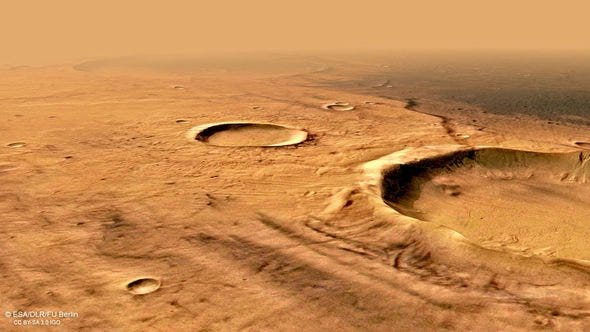# Anomaly Detection On Surface of Mars- Part 1 [Unsupervised Labeling on images for object detection…

Source: Deep Learning on Medium# Anomaly Detection On Surface of Mars- Part 1 [Unsupervised Labeling on images for object detection algorithms]

I have applied five levels of sharpening in my logic

From the example above

Level 1: More clarity on the image feature

Level 2: Better resolution that than level 1

Level 3: Started distorting the Image

Level 4: Destroyed a lot of features in the original image

Level 5: Almost all features are gone

## Summary: Original<level 1<Level2 ≤ Level3 >Level 4 > Level 5

Note: From here onward we will use level2 sharpening for all the images

# Thresholding:

Thresholding is the simplest operation in Image processing. The main idea is if a pixel value is above a certain threshold value assign it a particular value(say white) else assign it another value(say black).

## Note: Thresholding only takes grayscale images as input

Why Thesholding?

Thesholding will give as a image where regions of the image will be highlighted white and others parts as black that means the regions where there are objects will be white blob and rest will just become black. This gives us an clarity of presence of objects in the image.

Here I had designed the system for 2 types of threshold

1. Binary inverse threshold: Before Binary inverse threshold lets understand the concept of simple binary threshold, there are three parameters (source , threshold value, max value). If the source pixel value is greater than the threshold value then assign it max Value.

`if` `src(x,y) > thresh`

`dst(x,y) =` `maxValue`

`else`

`dst(x,y) =` `0`

Binary inverse is just the opposite to Binary threshold , if the source image pixel is greater than threshold assign it zero else assign it max value

`if` `src(x,y) > thresh`

`dst(x,y) =` `0`

`else`

`dst(x,y) =` `maxValue`

2. Zero Threshold: This method of threshold assigns source pixel to destination pixel if its greater than the threshold value else assigns zero.

`if` `src(x,y) > thresh`

`dst(x,y) =` `src(x,y)`

`else`

`dst(x,y) =` `0`

Comparing the two the Binary Inverse thresholding highlighted the Saturated areas and areas having some information within it be it small hills or creators.

ToZero thresholding Darkened those same regions.

FUN FACT: IF YOU COMBINE THIS TWO IMAGES USING ABSOLUTE DIFFERENCE YOU GET A EDGE DETECTOR

# Contour Detection /Shape detection:

Contours can be explained simply as a curve joining all the continuous points (along the boundary), having the same color or intensity. The contours are a useful tool for shape analysis and object detection and recognition.

** note: We are going to use rectangular Contour detection for this

The simple idea behind this is pixels with higher intensity forming a cluster can be identified. These Clusters are our regions of interests (The anomalies or objects in the image)

This is a two-step process:

Find Contours: this can be achieved by a simple function

cv2.findContours(ThresholdedImage,cv2.RETR_TREE,cv2.CHAIN_APPROX_NONE).

This will actually return coordinates for the detected points

Find the rectangular area bounding them: This can also be achived simply by using opencv

cv2.boundingRect(c)

where c is the contours we got ,this will return us width,height, x -coordinate and y-coordinate

This sounds familiar, right? this is exactly what is needed for algorithms like Yolo what they need is simply <xmax,xmin,ymax,ymin> and we have got those coordinates.

The above image looks clumsy, So what I finally did was to set up some parameters, like max dimension, max-width, min-width, max-height, min-height. This will limit the number of objects we get in the image.

As we can see if we set the parameters right we can limit the number of objects. For me, it was MaxDim that is width*height >1000 and or Min Height > 100 etc.